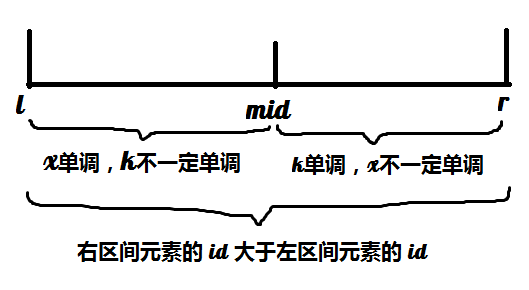# 浅谈斜率优化——截距优化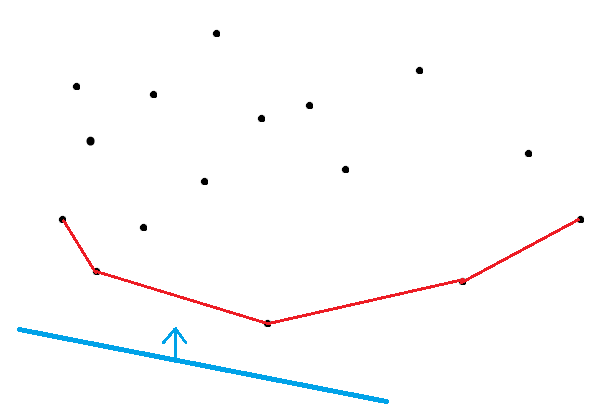• ### 1，$$x_j$$ 和 $$k_i$$ 均单调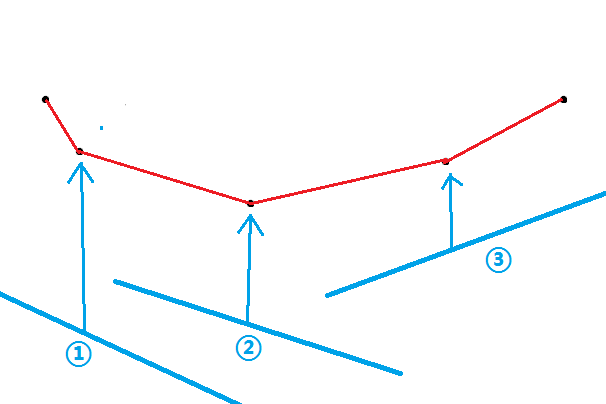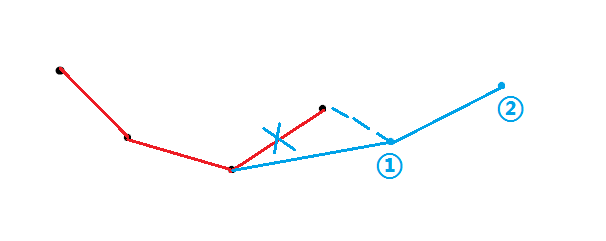• ### 2，只有 $$x_j$$ 单调

$$x_j$$ 是单调的，意味着仍然可以按照上面的方法加入一个点。那么查询呢？查询的 $$k_i$$ 不是单调的，这就意味着不能像之前一样弹出队头元素来找到最优点，因为最优点失去了一个性质——只会单调移动。（即这次的最优点可能在上一个最优点的左边。）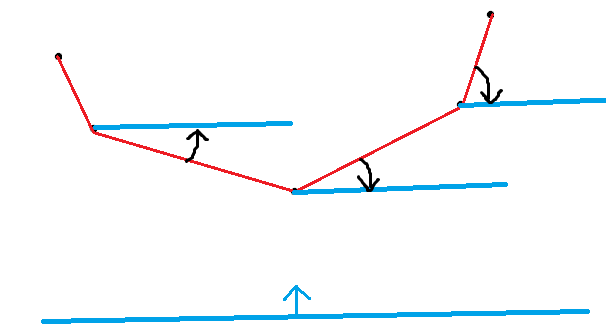• ### 3，$$x_j$$ 和 $$k_i$$ 都不单调